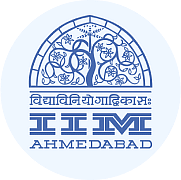CAT  >  Tricks for Fast Calculations

# Tricks for Fast Calculations Video Lecture - Quantitative Aptitude (Quant) - CAT

## Quantitative Aptitude (Quant)

179 videos|158 docs|113 tests

## FAQs on Tricks for Fast Calculations Video Lecture - Quantitative Aptitude (Quant) - CAT

 1. What are some tricks for fast calculations?Ans. Some tricks for fast calculations include using mental math techniques, breaking down complex problems into simpler steps, using shortcuts for multiplication and division, memorizing common multiplication tables, and practicing regularly to improve speed and accuracy.
 2. How can mental math techniques help in fast calculations?Ans. Mental math techniques involve performing mathematical calculations in your mind without the use of pen and paper or a calculator. These techniques help in performing calculations quickly and efficiently, saving time and effort. Mental math techniques include strategies such as using number patterns, estimation, rounding, and applying basic arithmetic rules.
 3. What are some shortcuts for multiplication and division?Ans. Some shortcuts for multiplication include using the distributive property, multiplying by powers of 10, and memorizing common multiplication tables. For division, shortcuts include using the concept of reciprocals, estimating the quotient, and canceling out common factors. These shortcuts can significantly speed up the calculation process.
 4. How can breaking down complex problems help in fast calculations?Ans. Breaking down complex problems into simpler steps helps in managing the calculations more effectively. By dividing a complex problem into smaller parts, it becomes easier to focus on each step and perform the calculations accurately. This approach also reduces the chances of making mistakes and allows for a faster calculation process.
 5. Why is regular practice important for improving calculation speed and accuracy?Ans. Regular practice is crucial for improving calculation speed and accuracy because it helps in building familiarity with different types of calculations and mathematical concepts. By practicing regularly, individuals become more comfortable with various mathematical operations, which leads to increased speed and reduced errors during calculations. Additionally, regular practice helps in reinforcing memory of multiplication tables, formulas, and other mathematical techniques.

## Quantitative Aptitude (Quant)

179 videos|158 docs|113 testsExplore Courses for CAT exam### How to Prepare for CAT

Read our guide to prepare for CAT which is created by Toppers & the best Teachers
Signup to see your scores go up within 7 days! Learn & Practice with 1000+ FREE Notes, Videos & Tests.
10M+ students study on EduRev
Track your progress, build streaks, highlight & save important lessons and more!
Related Searches

,

,

,

,

,

,

,

,

,

,

,

,

,

,

,

,

,

,

,

,

,

;